RENORMALIZED ENTANGLEMENT ENTROPY OF MASSDEFORMED ABJM THEORY Yong

• Slides: 40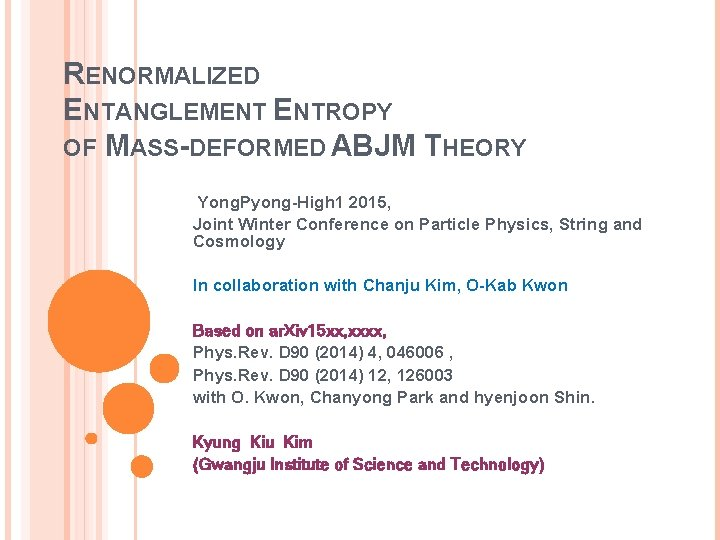RENORMALIZED ENTANGLEMENT ENTROPY OF MASS-DEFORMED ABJM THEORY Yong. Pyong-High 1 2015, Joint Winter Conference on Particle Physics, String and Cosmology In collaboration with Chanju Kim, O-Kab Kwon Based on ar. Xiv 15 xx, xxxx, Phys. Rev. D 90 (2014) 4, 046006 , Phys. Rev. D 90 (2014) 12, 126003 with O. Kwon, Chanyong Park and hyenjoon Shin. Kyung Kiu Kim (Gwangju Institute of Science and Technology)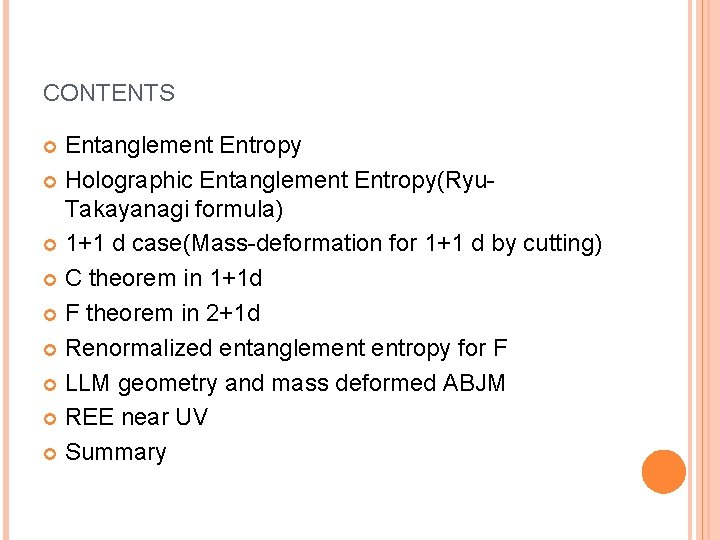CONTENTS Entanglement Entropy Holographic Entanglement Entropy(Ryu. Takayanagi formula) 1+1 d case(Mass-deformation for 1+1 d by cutting) C theorem in 1+1 d F theorem in 2+1 d Renormalized entanglement entropy for F LLM geometry and mass deformed ABJM REE near UV Summary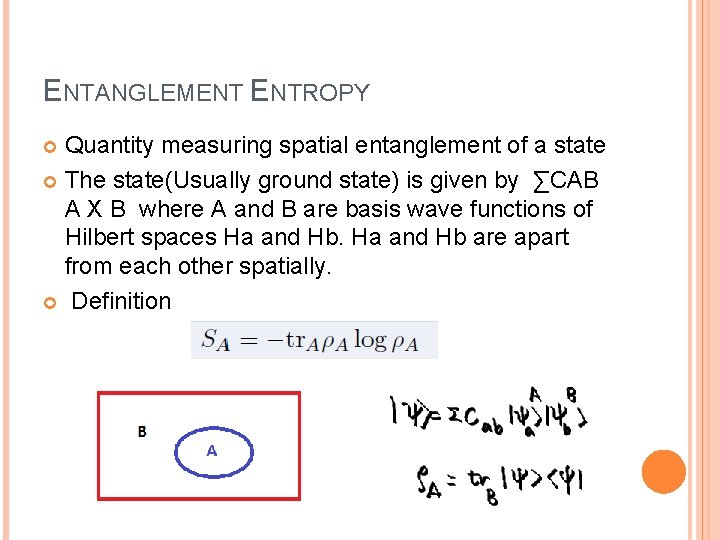ENTANGLEMENT ENTROPY Quantity measuring spatial entanglement of a state The state(Usually ground state) is given by ∑CAB A X B where A and B are basis wave functions of Hilbert spaces Ha and Hb are apart from each other spatially. DefinitionSimple example : two points and two spins Maximum entanglement entropy Minimum entanglement entropy with a trivial Hamiltonian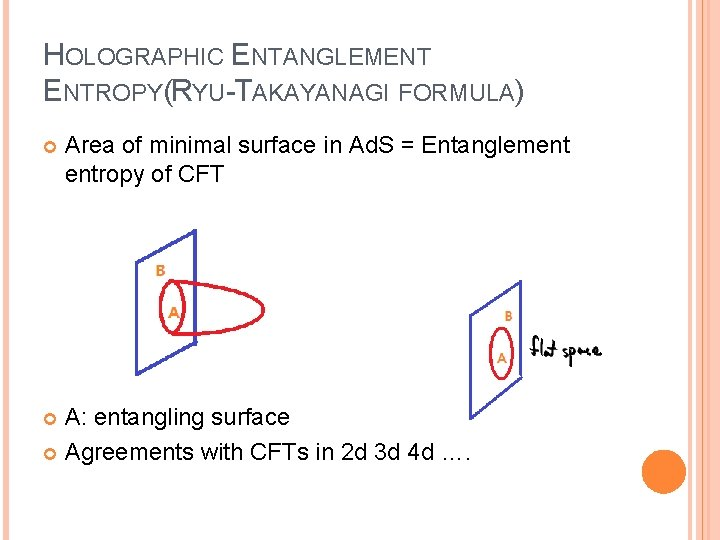HOLOGRAPHIC ENTANGLEMENT ENTROPY(RYU-TAKAYANAGI FORMULA) Area of minimal surface in Ad. S = Entanglement entropy of CFT A: entangling surface Agreements with CFTs in 2 d 3 d 4 d ….From the field theories(2 dimensions and higher dimensions Minimal surfaces have same structure of these UV divergences.1+1 D CASE From field theory 94’ Minimal surface(geodesic) in Ad. S 3 Mass deformation C. Holzhey, F. Larsen and F. Wilczek,Computation through HEE = log ( tan s/2 )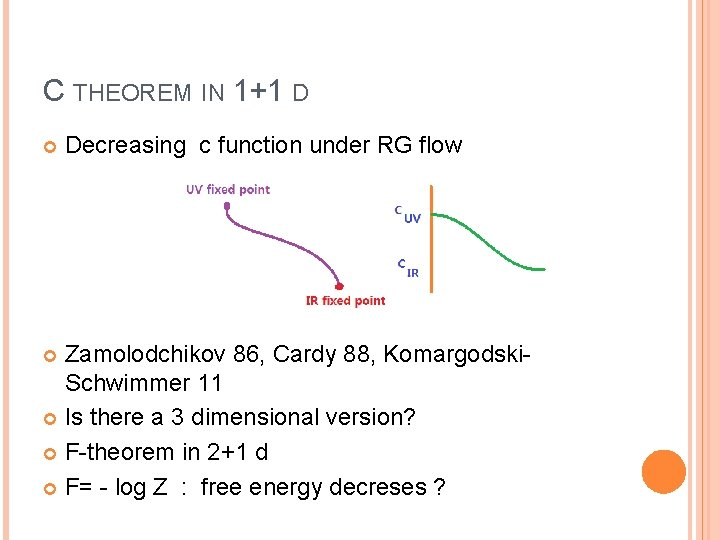C THEOREM IN 1+1 D Decreasing c function under RG flow Zamolodchikov 86, Cardy 88, Komargodski. Schwimmer 11 Is there a 3 dimensional version? F-theorem in 2+1 d F= - log Z : free energy decreses ?F theorem (Jafferis-Klebanov-Pufu-Safdi 11, Myers-Sinha 10 ) F_UV > F_IR For CFT_3 [Casini-Huerta-Myers 11 ]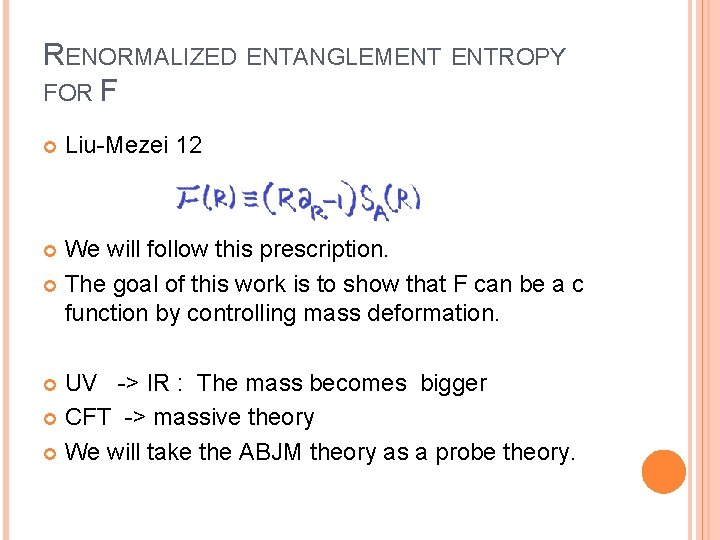RENORMALIZED ENTANGLEMENT ENTROPY FOR F Liu-Mezei 12 We will follow this prescription. The goal of this work is to show that F can be a c function by controlling mass deformation. UV -> IR : The mass becomes bigger CFT -> massive theory We will take the ABJM theory as a probe theory.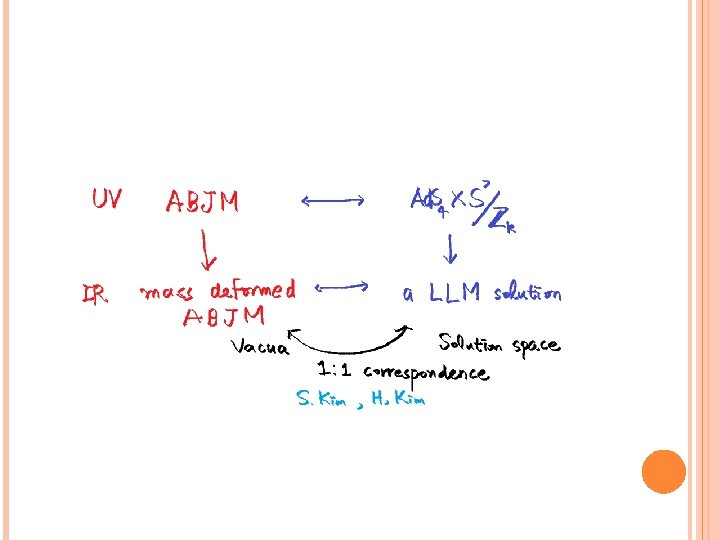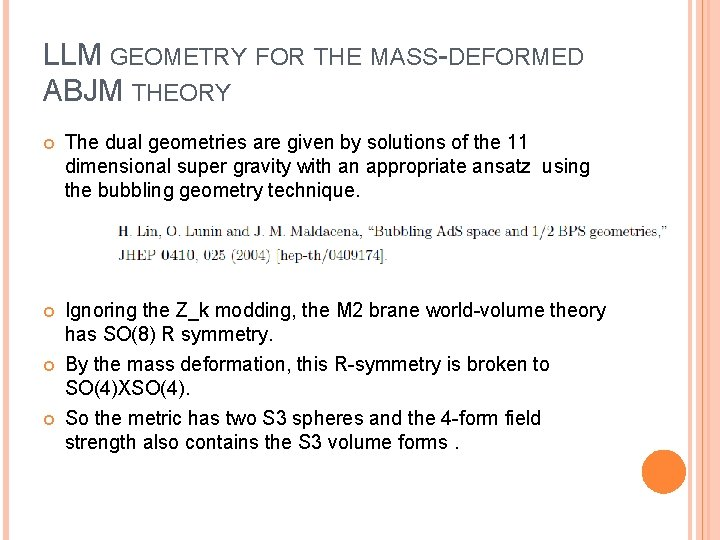LLM GEOMETRY FOR THE MASS-DEFORMED ABJM THEORY The dual geometries are given by solutions of the 11 dimensional super gravity with an appropriate ansatz using the bubbling geometry technique. Ignoring the Z_k modding, the M 2 brane world-volume theory has SO(8) R symmetry. By the mass deformation, this R-symmetry is broken to SO(4)XSO(4). So the metric has two S 3 spheres and the 4 -form field strength also contains the S 3 volume forms.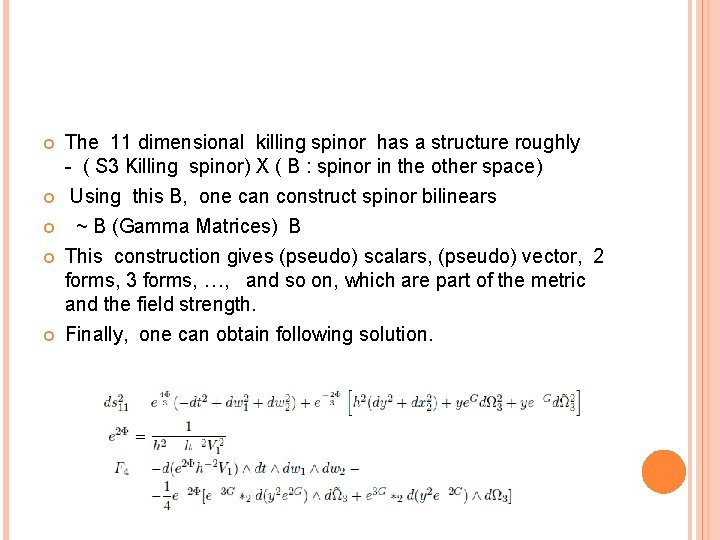The 11 dimensional killing spinor has a structure roughly - ( S 3 Killing spinor) X ( B : spinor in the other space) Using this B, one can construct spinor bilinears ~ B (Gamma Matrices) B This construction gives (pseudo) scalars, (pseudo) vector, 2 forms, 3 forms, …, and so on, which are part of the metric and the field strength. Finally, one can obtain following solution.Considering Z_k modding and SO(2, 1) isometry to the Ansatz give the solution we will consider as follows.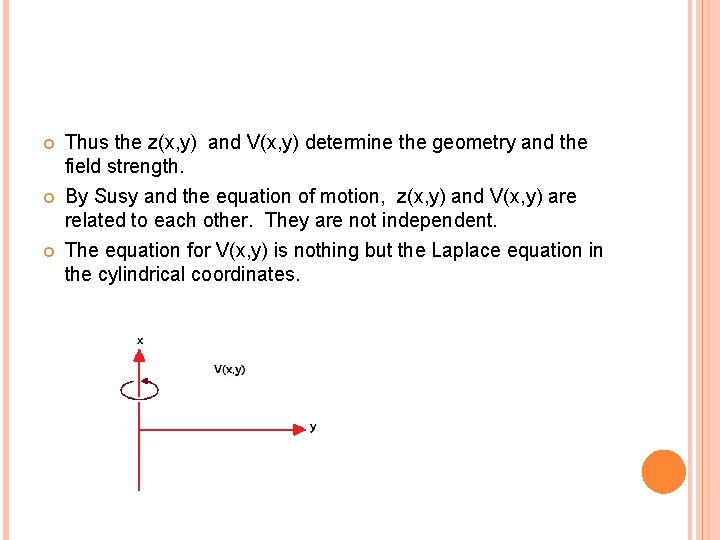Thus the z(x, y) and V(x, y) determine the geometry and the field strength. By Susy and the equation of motion, z(x, y) and V(x, y) are related to each other. They are not independent. The equation for V(x, y) is nothing but the Laplace equation in the cylindrical coordinates.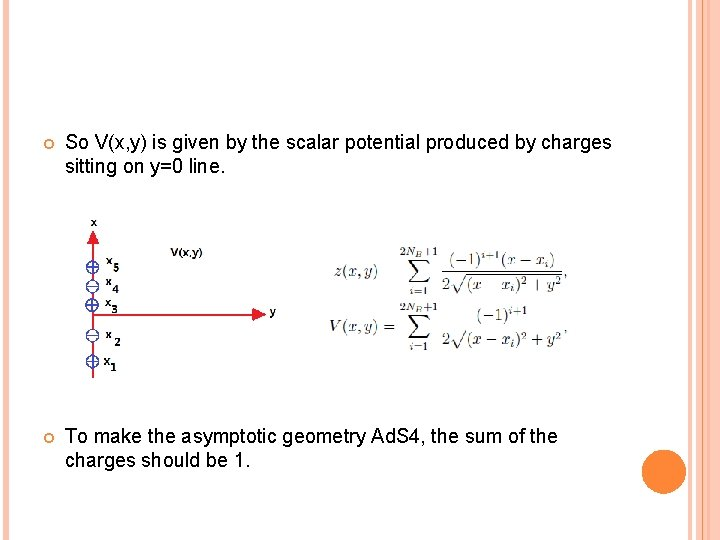So V(x, y) is given by the scalar potential produced by charges sitting on y=0 line. To make the asymptotic geometry Ad. S 4, the sum of the charges should be 1.DISCRETE VACUA FOR THE MASS-DEFORMED ABJM THEORY There are infinite number of discrete vacua encoded by VEV of the scalar field. GRVV matrix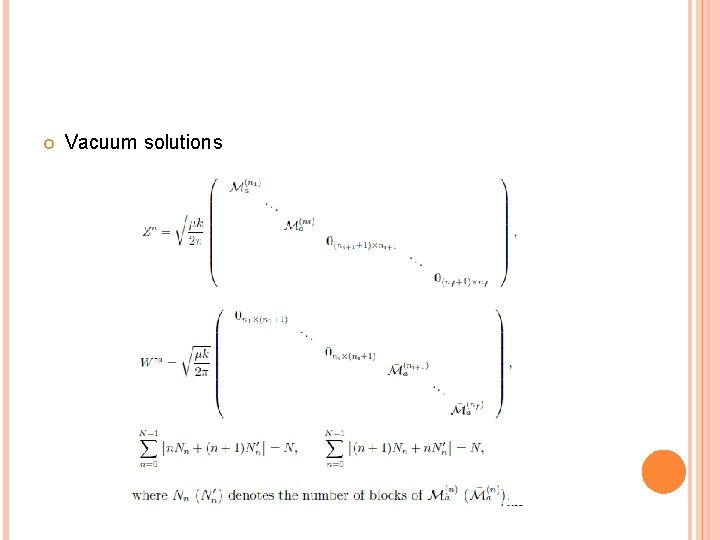Vacuum solutions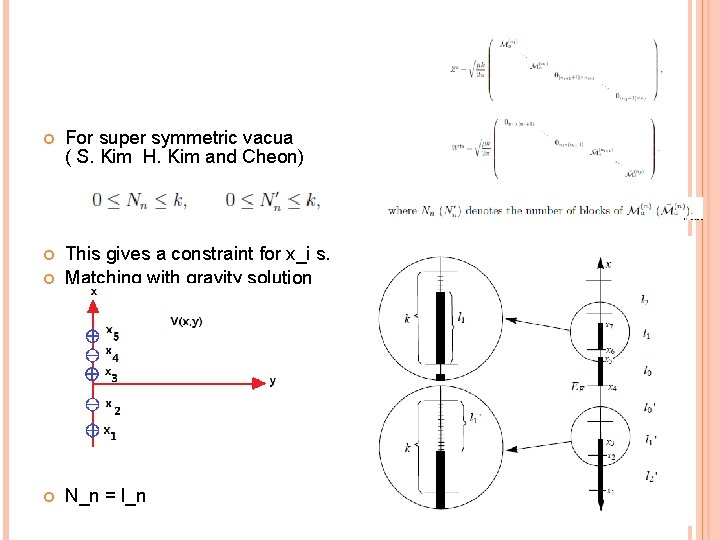For super symmetric vacua ( S. Kim H. Kim and Cheon) This gives a constraint for x_i s. Matching with gravity solution N_n = l_n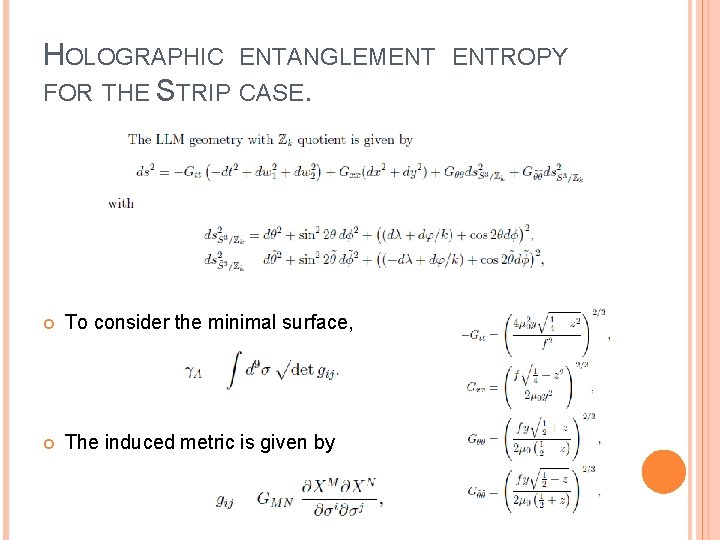HOLOGRAPHIC ENTANGLEMENT FOR THE STRIP CASE. To consider the minimal surface, The induced metric is given by ENTROPY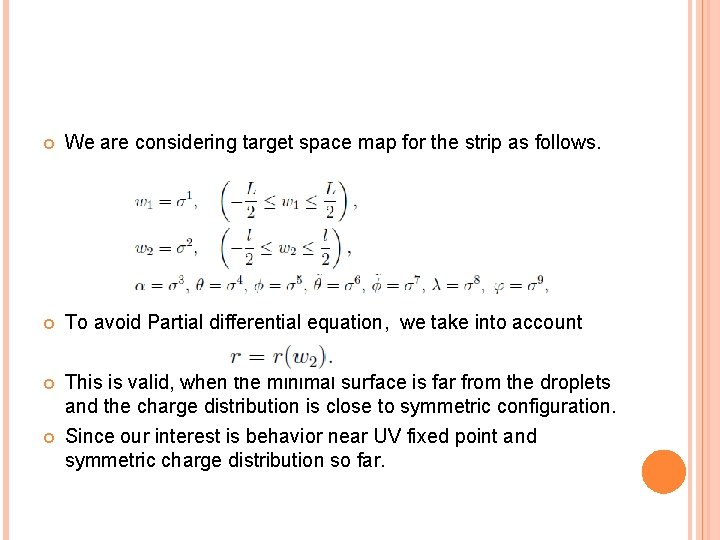We are considering target space map for the strip as follows. To avoid Partial differential equation, we take into account This is valid, when the minimal surface is far from the droplets and the charge distribution is close to symmetric configuration. Since our interest is behavior near UV fixed point and symmetric charge distribution so far.The minimal surface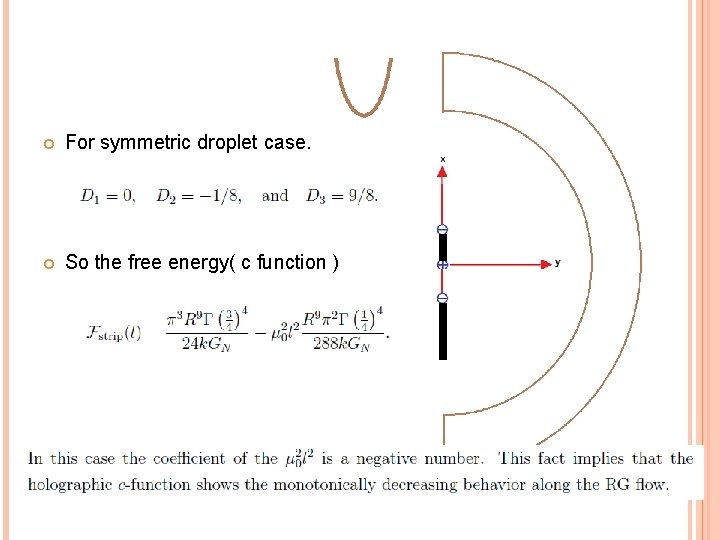For symmetric droplet case. So the free energy( c function )HOLOGRAPHIC ENTANGLEMENT FOR THE DISK CASE. The target space map is given by The minimal surface Lagrangian is In the small mass approximation ENTROPYSubstituting l, the minimal surface, the holographic entanglement for the strip case. The renormalized entanglement entropy is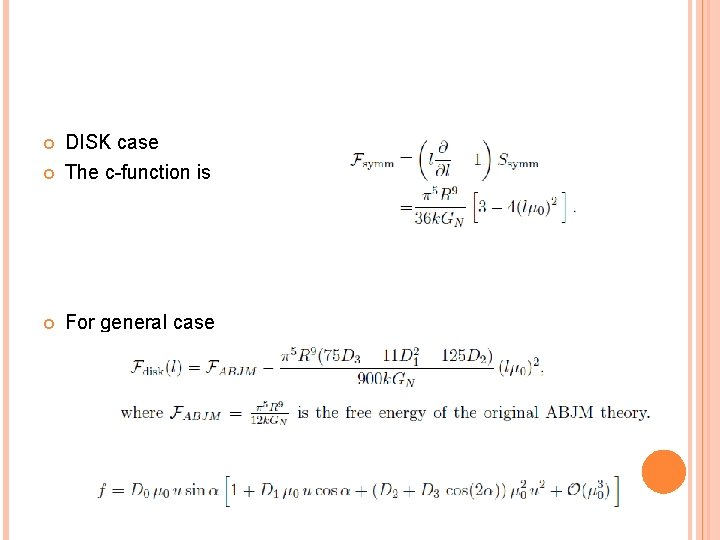DISK case The c-function is For general caseBecause of the Ad. S metric factor, the minimal surface has a counter-intuitive shape as follows.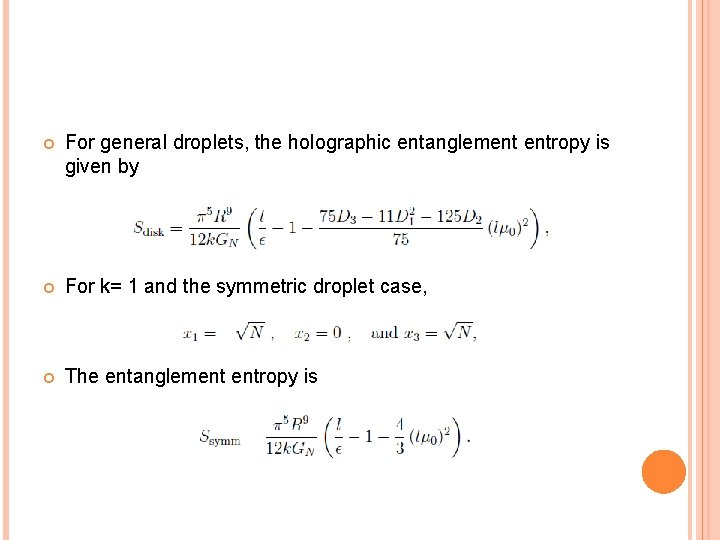For general droplets, the holographic entanglement entropy is given by For k= 1 and the symmetric droplet case, The entanglement entropy isThe c-function is For general caseFor the symmetric case, the c-function or the partition function shows the monotonic decreasing behavior. For the general case, it is not guaranteed. We need to investigate the validity of our computation and the modification of the coefficient. ?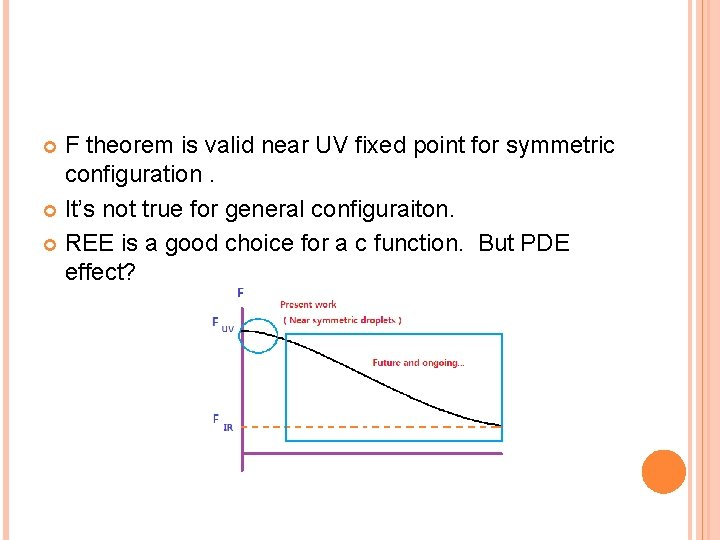F theorem is valid near UV fixed point for symmetric configuration. It’s not true for general configuraiton. REE is a good choice for a c function. But PDE effect?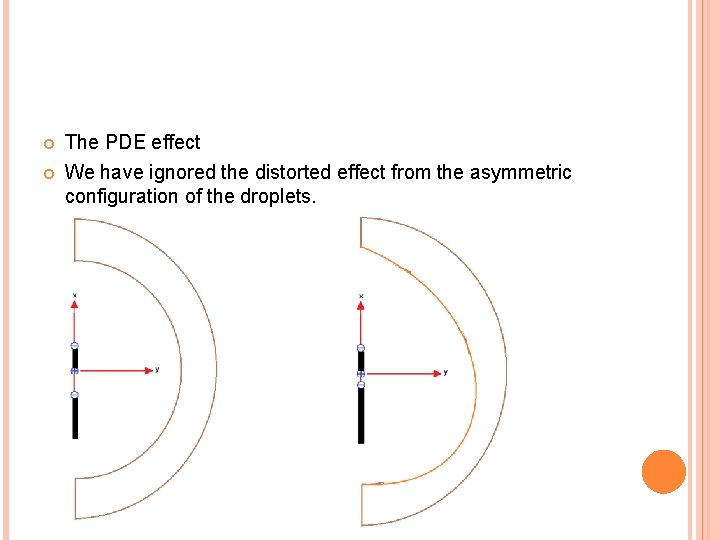The PDE effect We have ignored the distorted effect from the asymmetric configuration of the droplets.FOR PDE EFFECT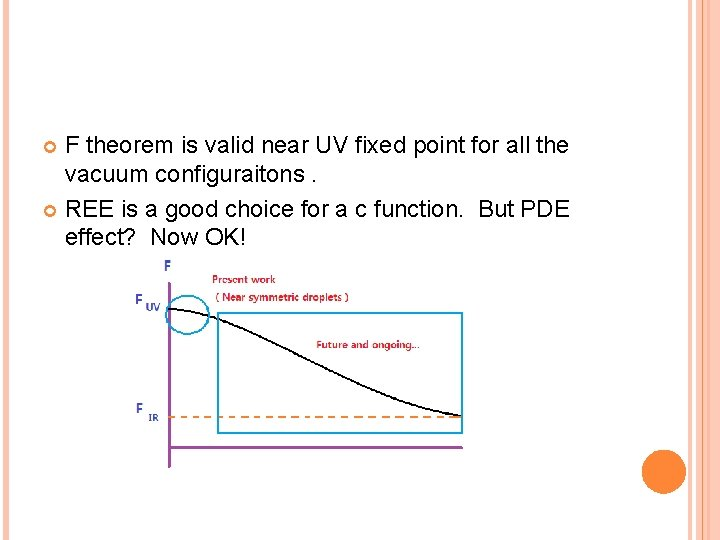F theorem is valid near UV fixed point for all the vacuum configuraitons. REE is a good choice for a c function. But PDE effect? Now OK!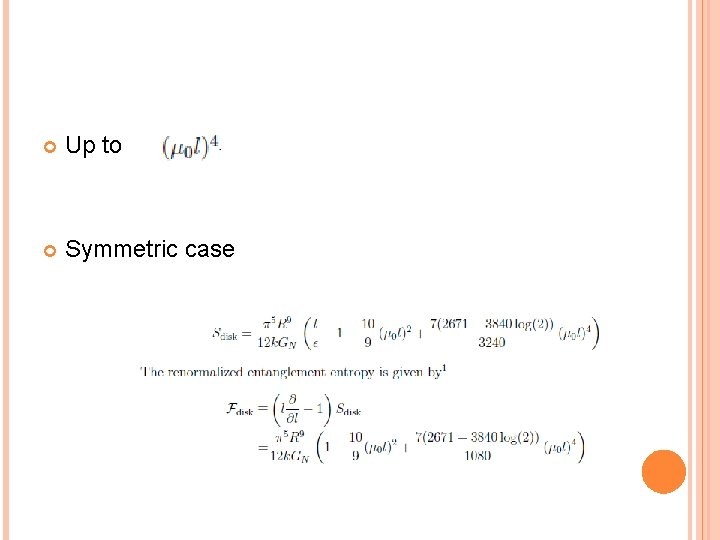Up to Symmetric case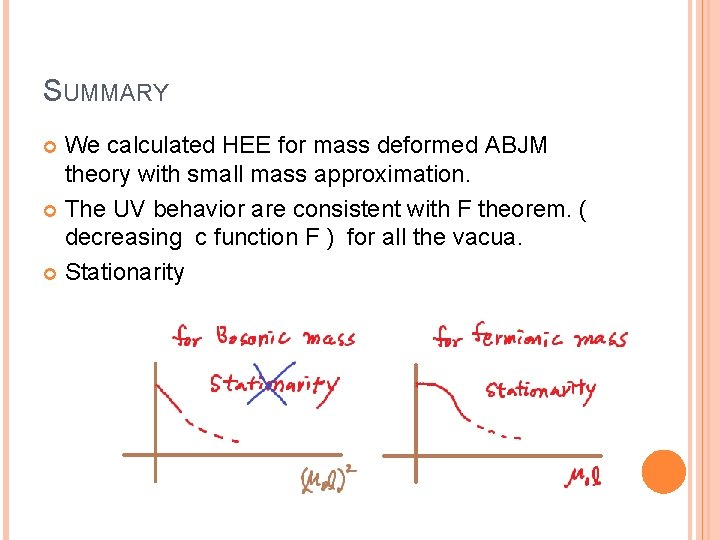SUMMARY We calculated HEE for mass deformed ABJM theory with small mass approximation. The UV behavior are consistent with F theorem. ( decreasing c function F ) for all the vacua. Stationarity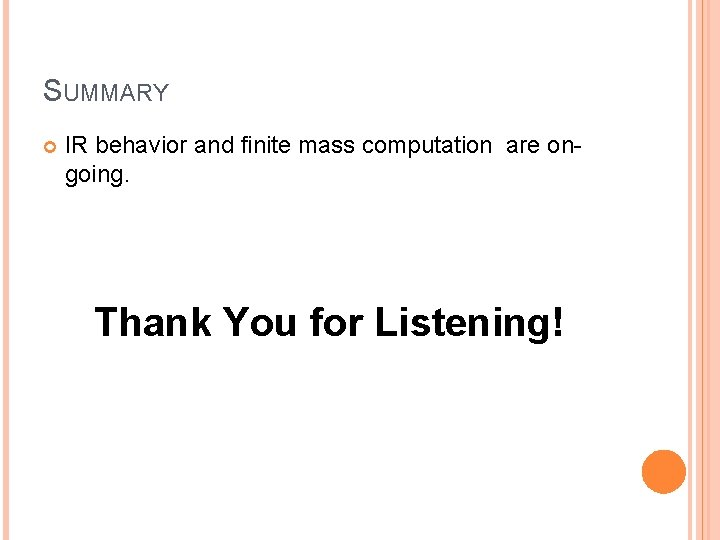SUMMARY IR behavior and finite mass computation are ongoing. Thank You for Listening!